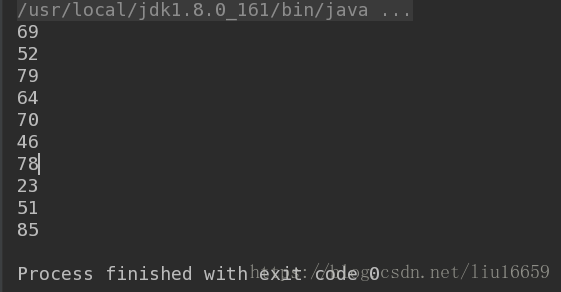# 使用Scala生成随机数的方法示例

更新时间：2019年06月16日 15:13:21   作者：LittleLawson

1.简单版本：

```/*
1.you can use scala.util.Random.nextInt(10) to produce a number between 1 and 10
2.at the same time,you nextInt(100) to produce a number between 1 and 100
*/
object Test {
def main(args: Array[String]) {
var i = 0
while(i < 10)
var str = scala.util.Random.nextInt(100).toString
println(str)
i = i+1
}
}
}```2.复杂版本：

```object Test{
def main(args: Array[String]): Unit = {
val wordPerMessage = 4
var i = 0
while(i<10){
/*
1.the (1 to 1) is meaning that only have one circulation.
*/
(1 to 1).foreach { messageNum => {
//[There's only three cycle]
val str: Seq[String] = (1 to wordPerMessage).map(x => scala.util.Random.nextInt(10).toString)
val str1 = str.mkString(" ")//separate str1 with space
println(str)
}
}
i = i +1
}
}
}```

PS:scala生成一组不重复的随机数

1、循环获取随机数，再到 list中找，如果没有则添加

```def randomNew(n:Int)={
var resultList:List[Int]=Nil
while(resultList.length<n){
val randomNum=(new Random).nextInt(20)
if(!resultList.exists(s=>s==randomNum)){
resultList=resultList:::List(randomNum)
}
}
resultList
}```

2、每次生成一个随机数index，将index作为数组下标取相应的元素，然后去除该元素，下一次生成随机数的范围减1，

```def randomNew2(n:Int)={
var arr= 0 to 20 toArray
var outList:List[Int]=Nil
var border=arr.length//随机数范围
for(i<-0 to n-1){//生成n个数
val index=(new Random).nextInt(border)
println(index)
outList=outList:::List(arr(index))
arr(index)=arr.last//将最后一个元素换到刚取走的位置
arr=arr.dropRight(1)//去除最后一个元素
border-=1
}
outList
}

```